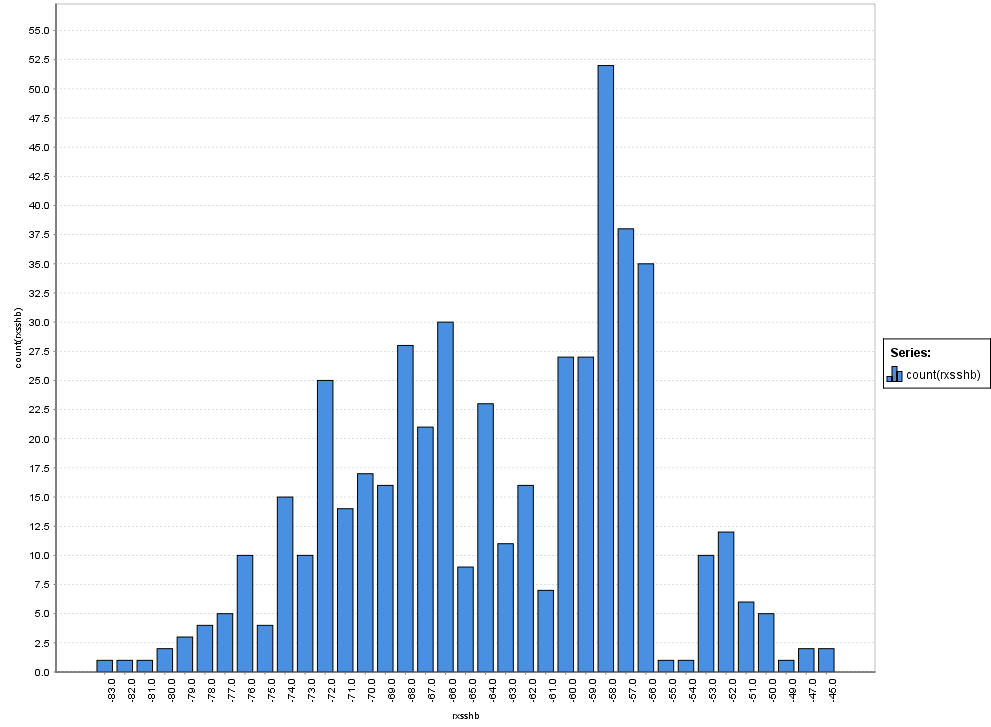# creating 2 histogreams on 1 chart in advanced charts

Member Posts: 6Contributor I
edited December 2018 in Help

I have a output data set that looks something like this;

 row item mac add loc rxsslb rxsshb txsslb txsshb freqhb freqlb 1 3 e8:4e:06:49:3e:cf wlan0 -57 -68 -46 -59 5240 2437

where all the columns except "mac add" are intergers

I would like two (or more) columns on a common histogram (lets take rxsshb, and txsshb for this example) with bins at each descrete value, i.e. -50, -51, and so on. In other words I want the population of each value of rxsshb and txsshb to be visualized. in bars whose height is the population, and color is the column (rxsshb/txsshb), translucent appearing (so I can see overlay)

The funny thing if the basic chart does this "pretty good" by simply ctrl-left-click the second column name in the dialog, BUT....the bins seem to be "offset" a little.

When I try this in advanced, by assigning rxsshb to the domain dimension, and count(rxsshb) to the numerical axis, and setting everything appropriate to give me catagorized histogram, its perfect, but I cannot add the second set of values (txsshb) to the chart. Am I stuck using the basic chart for this?

cmosesTagged:

• Moderator, RapidMiner Certified Analyst, Member Posts: 1,195Unicorn

Hi @cmosesjr,

Indeed it seems that there is problem with multiple series in advanced charts.

So I propose a (far-fetched) paliative solution to build your chart :

First I assume that your dataset is under this form :We suppose that you want to represent "rxsslb" and "rxsshb" :

Add a De-Pivot operator after the operator which generate your dataset and in the parameters of this operator :

- set attribute name like that :- set the name of index attribute (in my example "index") :Execute the process and go to the "Advanced Charts" panel and set your chart like that :

- Domain dimension : numbers

- Color dimension : index

- Numerical axis : count(index)

You obtain a chart like that :Note :  There is a display bug, there is a "shift" of the bars in the X-axis (for example the first bar, to the left, is at -75 instead of -80).

I hope it helps,

Regards,

Lionel

NB2 : The process :

`<?xml version="1.0" encoding="UTF-8"?><process version="9.0.001">  <context>    <input/>    <output/>    <macros/>  </context>  <operator activated="true" class="process" compatibility="9.0.001" expanded="true" name="Process">    <process expanded="true">      <operator activated="true" class="read_excel" compatibility="9.0.001" expanded="true" height="68" name="Read Excel" width="90" x="112" y="85">        <parameter key="excel_file" value="C:\Users\Lionel\Documents\Formations_DataScience\Rapidminer\Tests_Rapidminer\Adv_Charts\Adv_Charts.xlsx"/>        <list key="annotations"/>        <parameter key="date_format" value="MMM d, yyyy h:mm:ss a z"/>        <list key="data_set_meta_data_information">          <parameter key="0" value="row.true.integer.attribute"/>          <parameter key="1" value="item.true.integer.attribute"/>          <parameter key="2" value="mac add.true.polynominal.attribute"/>          <parameter key="3" value="loc.true.polynominal.attribute"/>          <parameter key="4" value="rxsslb.true.integer.attribute"/>          <parameter key="5" value="rxsshb.true.integer.attribute"/>          <parameter key="6" value="txsslb.true.integer.attribute"/>          <parameter key="7" value="txsshb.true.integer.attribute"/>          <parameter key="8" value="freqhb.true.integer.attribute"/>          <parameter key="9" value="freqlb.true.integer.attribute"/>        </list>        <parameter key="read_not_matching_values_as_missings" value="false"/>      </operator>      <operator activated="true" class="de_pivot" compatibility="9.0.001" expanded="true" height="82" name="De-Pivot" width="90" x="313" y="85">        <list key="attribute_name">          <parameter key="numbers" value="rx.*"/>        </list>        <parameter key="index_attribute" value="index"/>      </operator>      <connect from_op="Read Excel" from_port="output" to_op="De-Pivot" to_port="example set input"/>      <connect from_op="De-Pivot" from_port="example set output" to_port="result 1"/>      <portSpacing port="source_input 1" spacing="0"/>      <portSpacing port="sink_result 1" spacing="0"/>      <portSpacing port="sink_result 2" spacing="0"/>    </process>  </operator></process>`